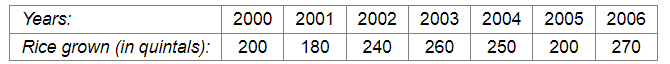# The following table shows the amount of rice grown by a farmer in different years:

Question:

The following table shows the amount of rice grown by a farmer in different years:Plot a graph to illustrate this information.

Solution:

Here, year is an independent variable and quantity of rice grown is a dependent variable. So, we take years on the x-axis and quantity of rice grown on the y-axis.

Let us choose the following scale:

On x-axis: 2 cm = 1 year

On y-axis: 1 cm = 20 quintals

Let us assume that the origin O represents the coordinates (1999, 160).

Now, let us plot (2000, 200), (2001, 180), (2002, 240), (2003, 260), (2004, 250),(2005, 200),(2006, 270). These points are joined to get the graph representing the given information as shown in the figure below.​